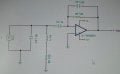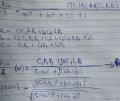# Derivation of RLC parameters from a complex RLC circuit

#### kwoki

Joined Aug 31, 2018
10
Hey guys,

I am having trouble with a problem. I would like to derive a formula for some parameters (resonance frequency, Quality factor, Wcl and Wch)
Normally, you will use a general second-order function for a second-order RLC circuit (which is easy to derive). However, my circuit is much more complex and is a third-order transfer function.

Besides, the values are just random picked.

So does anyone how to solve it? (It's actually not a homework, it's a problem which is related to a project of mine)

The circuitThe derived Transfer functionregards,
Kwoki

#### RBR1317

Joined Nov 13, 2010
693
So does anyone how to solve it? (It's actually not a homework, it's a problem which is related to a project of mine)
In order to analyze the circuit one would need to solve the node equations. In this case the two node voltages will be the input voltage Vi across the current source, and the (-) input to the op-amp which will always be equal to the (+) input, i.e. zero. Neither of these voltages have much significance but will allow for solution of Vtia/I.
(Note: This took about 2 minutes to solve; but, not sure about posting solutions in the Homework Help for problems that are not homework.)

#### kwoki

Joined Aug 31, 2018
10
In order to analyze the circuit one would need to solve the node equations. In this case the two node voltages will be the input voltage Vi across the current source, and the (-) input to the op-amp which will always be equal to the (+) input, i.e. zero. Neither of these voltages have much significance but will allow for solution of Vtia/I.
(Note: This took about 2 minutes to solve; but, not sure about posting solutions in the Homework Help for problems that are not homework.)
Thank you for your reply, But The transfer function of Vtia/I is already done (The derived transferfunction of the Vtia/I picture; it's also verified) That's the transfer function of that circuit. My real question is How to derive some formulas for resonance frequency and Q-factor as parameters from the transfer function.

#### MrAl

Joined Jun 17, 2014
9,157
Hello,

One way to solve for resonance (the max or min amplitude type) is to take the derivative of the amplitude of the transfer function with respect to w and then find the w where the max (or min) occurs. Once you have the wo for max response you insert that back into the transfer function to find the center gain Ao.
Now that you have the center frequency wo and gain Ao, you solve the amplitude response for the two -3db points using:
p=Ao/sqrt(2)
at two different frequencies w1 and w2.
The bandwidth is then:
BW=w2-w1
and so the Q is:
Q=wo/BW

So mathematically the transfer function:
Ts(w)
calculate the amplitude A(w) then the derivative looks like this:
dA(w)/dw
set that equal to zero:
dA(w)/dw=0
solve for the center frequency wo, then calculate:
Ao=A(wo)
then solve A(w) for the two points from:
A=Ao/sqrt(2)
and that gives you two frequencies w1 and w2 then the bandwidth is as above and the Q is as above.

This method ensures you find the center frequency that results in the maximum (or minimum) amplitude. This is usually the way this class of circuit is done rather than calculate the physical resonance, which sometimes could be the same though.

If it is low pass or high pass then there will only be one -3db point not two.

This circuit looks like a pseudo LP because of low Q but does have a peak.

Last edited:

#### RBR1317

Joined Nov 13, 2010
693
How to derive some formulas for resonance frequency and Q-factor as parameters from the transfer function.
Here is the example of letting the computer do the work....

#### Attachments

• 153.4 KB Views: 4
Last edited:

#### kwoki

Joined Aug 31, 2018
10
Here is the example of letting the computer do the work....
Thank your for your input. Ah, I didn't know that you can plot the bodeplot with Maple.

#### kwoki

Joined Aug 31, 2018
10
Hello,

One way to solve for resonance (the max or min amplitude type) is to take the derivative of the amplitude of the transfer function with respect to w and then find the w where the max (or min) occurs. Once you have the wo for max response you insert that back into the transfer function to find the center gain Ao.
Now that you have the center frequency wo and gain Ao, you solve the amplitude response for the two -3db points using:
p=Ao/sqrt(2)
at two different frequencies w1 and w2.
The bandwidth is then:
BW=w2-w1
and so the Q is:
Q=wo/BW

So mathematically the transfer function:
Ts(w)
calculate the amplitude A(w) then the derivative looks like this:
dA(w)/dw
set that equal to zero:
dA(w)/dw=0
solve for the center frequency wo, then calculate:
Ao=A(wo)
then solve A(w) for the two points from:
A=Ao/sqrt(2)
and that gives you two frequencies w1 and w2 then the bandwidth is as above and the Q is as above.

This method ensures you find the center frequency that results in the maximum (or minimum) amplitude. This is usually the way this class of circuit is done rather than calculate the physical resonance, which sometimes could be the same though.

If it is low pass or high pass then there will only be one -3db point not two.

This circuit looks like a pseudo LP because of low Q but does have a peak.
Thank your for your explanation. I will try it and I hope this will take me a step closer

#### MrAl

Joined Jun 17, 2014
9,157
Thank your for your explanation. I will try it and I hope this will take me a step closer
Ok sure you are welcome. If you have problems just post here.
I know it wont be easy because this is third order, but it is still very possible to do. I went through i once just to make sure.

#### kwoki

Joined Aug 31, 2018
10
Ok sure you are welcome. If you have problems just post here.
I know it wont be easy because this is third order, but it is still very possible to do. I went through i once just to make sure.
Well, I am still stuck with calculations of the center frequency, because matlab won't calculate the formula for my w0 from the differentiated formula (which is equal to 0 for no gradient) of the absolute transfer function.

I have attached a rar with matlab file and a picture inside to let you see what I have done in matlab.
If this is not going to work, is it the best to do another approach like RBR1317 said by just using the bodeplot to analyze it without calculations?

#### Attachments

• 39.7 KB Views: 1

#### MrAl

Joined Jun 17, 2014
9,157
OH sorry to hear that.
But yes you can use a frequency response plot in a simulator and try to zoom in on the peak, and that will get you the center frequency.
When doing the math there may be a few tricks you have to apply to the resulting diff expression. For example, if you do part of it manually like square both sides and multiply out the denominator (that goes away when there is a zero on the right) but you may have to factor first. I know for sure it is possible because i have done it myself and obtained the center frequency as well as the other frequencies.
I dont have Matlab though so not sure how much help i can be there. I use Maxima cause it is free.
If you want to use a simulator and pick the results from that, you can provide me with your results and i will compare them to the exact calculations.

#### kwoki

Joined Aug 31, 2018
10
I have attached my calculations, bodeplot and where I tried to equal the derivated formula to 0, but got some errors I don't know.
Furthermore, I have also calculated the gain using w0 = 4.86e4 rad/sec (bodeplot) to verify the transfer function. It seems correct.

Maybe in maxima it's more easier to get the derivated formula from the |TIA/I|?? I have never used it before

#### MrAl

Joined Jun 17, 2014
9,157
Ok well do you know how to calculate or find the two -3db points?
If you find them F1 and F2 then you can use center frequency of (F1+F2)/2.
If you dont mind being approximate you can use any part of the flat midband portion as reference in finding the two -3db points.

It looks like 10600 is a good amplitude to use as the midband gain at around 1e6Hz.

It is interesting that i get a different peak frequency than you did, i guess that is because of the op amp parasitics vs pure theory. In pure theory the op amp is ideal.

Last edited: